• 利用 AUC（ROC 曲线下的面积）为评价指标，结合单类 F-score 特征选择和遗传算法建立多层神经网络模型，选出对于不平衡数据分类更有利的特征子集，从而建立更适用于不平衡数据分类的深度模型。基于Tensor Flow建立...不平衡数据 遗传算法
• MNIST手写数字识别问题的多层神经网络模型实践 按课程案例，动手完成编码实践。 自行设计一种神经网络模型，并尝试采用不同超参数，让模型的准确率达到97.5%。 提交要求： 1、你认为最优的一次带运行结果的源代码...
• 深度学习原理及应用 介绍了卷积神经网络 自动编码器等基础知识的ppt, 深度学习本质上是层次特征提取学习的过程， 它通过构建多层隐含神经网络模型， 利用海量数据训练出模型特征来提取最有利的参数， 将简单的特征...深度学习
• MLP（多层感知器神经网络）即多层全连接神经网络模型。 from keras.datasets import mnist from keras.utils import np_utils from keras.models import Sequential from keras.layers import Dense,Dropout,...
MLP（多层感知器神经网络）即多层全连接神经网络模型。
from keras.datasets import mnist
from keras.utils import np_utils
from keras.models import Sequential
from keras.layers import Dense,Dropout,Activation

batch_size=128
nb_classes=10
nb_epoch=10
img_size=28*28

x_train=x_train.reshape(-1,img_size).astype('float32')/255
x_test=x_test.reshape(-1,img_size).astype('float32')/255
y_train=np_utils.to_categorical(y_train,nb_classes)
y_test=np_utils.to_categorical(y_test,nb_classes)

model=Sequential([
Dense(512,input_shape=(img_size,),activation='relu',),
Dropout(0.2),
Dense(512,input_shape=(512,),activation='relu'),
Dropout(0.2),
Dense(10,input_shape=(512,),activation='softmax'),
])

model.summary()

model.compile(optimizer='rmsprop',loss='categorical_crossentropy',metrics=['accuracy'])

model.fit(x_train,y_train,batch_size=batch_size,epochs=10,verbose=0,validation_data=(x_test,y_test))

score=model.evaluate(x_test,y_test,verbose=0)
print('accuracy:'+str(score))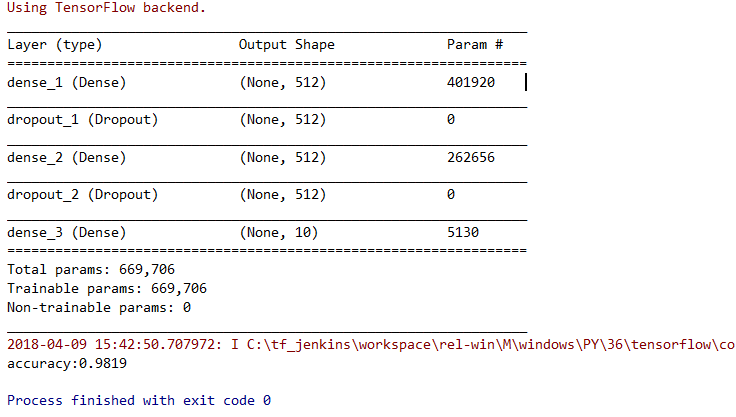展开全文• c++实现多层神经网络模型，工程用vs2008生成。 参考了网上多篇c++神经网络代码，修改多处bug，最终生成无bug版本的代码 测试例子为自己构造：将3位二进制转为10进制
• 2）多层神经网络模型 3）区别 二、三层神经网络 1、定理 2、一些概念（决策面、神经元、神经网络层数） 1）决策面 2）神经元 3）神经网络层数n 3、常见的三层神经网络模型（含w,b的参数设置） 1）一个...
目录
一、多层神经网络
1、多层神经网络数学模型
2、数学模型中的非线性函数fai
1）非线性函数fai存在的意义
2）非线性函数fai具体是什么？
3、多层神经网络与单层神经网络的区别与改进
1）单层神经网络数学模型
2）多层神经网络模型
3）区别
二、三层神经网络
1、定理
2、一些概念（决策面、神经元、神经网络层数）
1）决策面
2）神经元
3）神经网络层数n
3、常见的三层神经网络模型（含w,b的参数设置）
1）一个三角形决策面的神经网络模型（两层神经网络）
2）一个四边形决策面的神经网络模型（两层神经网络）
3）一个曲线围成决策面的神经网络模型（两层神经网络）
小结1：一个决策面时最后一层常用w,b参数的设置
4)两个决策面的神经网络模型（三层神经网络）
5)两个以上决策面的神经网络模型（三层神经网络）
小结2：多个决策面时最后一层w,b常用参数和第二层w,b常用参数的设置
疑问：

一、多层神经网络
1、多层神经网络数学模型
并行化的系统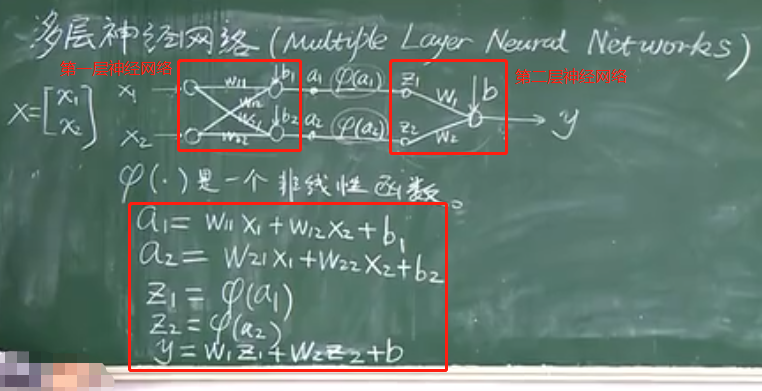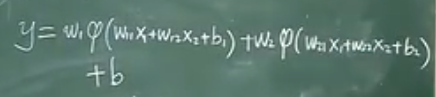2、数学模型中的非线性函数fai
1）非线性函数fai存在的意义
如下图可知如果没有非线性函数最后得出的y依据是线性的，这就又回到了最初的单层神经网络，没有新的突破，而fai函数则图突破了这个局限性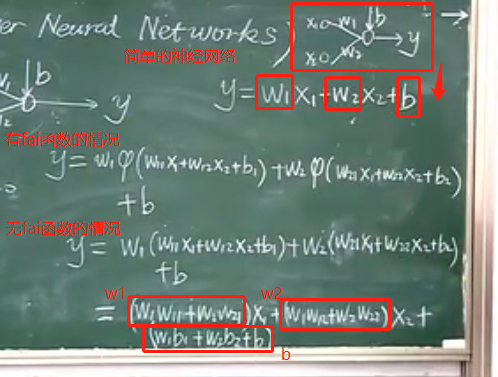2）非线性函数fai具体是什么？
fai函数就是阶跃函数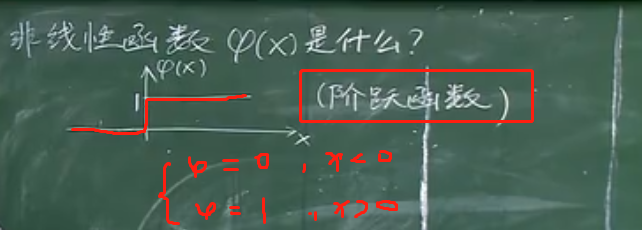3、多层神经网络与单层神经网络的区别与改进
1）单层神经网络数学模型
具体见：《机器学习——人工神经网络之发展历史（神经元数学模型、感知器算法）》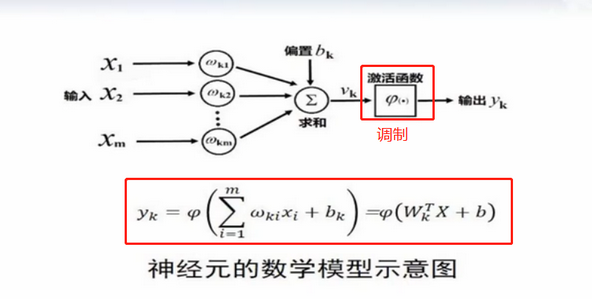2）多层神经网络模型3）区别
多层神经网络顾名思义具有多层的神经网络，经过多层的变化后将输入的xi变化后进行y的输出
该多层神经网络数学模型和阶跃函数（fai函数）的结合可以解决所有的非线性问题，这也是多层神经网络最大的优势所在

二、三层神经网络
1、定理
三层神经网络可以模拟所有的决策面
2、一些概念（决策面、神经元、神经网络层数）
1）决策面
决策面就是每一类样本所分布的一块区域，由多条线所围成的一个区域（若由曲线围成，可以将曲线看成由无数条非常短的线组成的曲线）
三条线围成一个决策面（C1类的区域）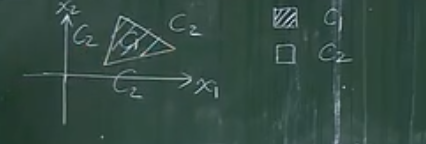四条线围成一个决策面（C1类的区域）曲线围成一个决策面（C1类的区域）——将曲线看成由无数直线构成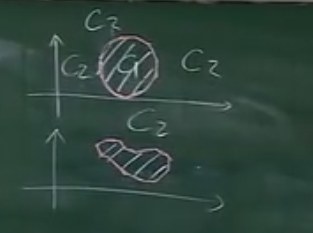直线围成多个分开的决策面（C1类的区域）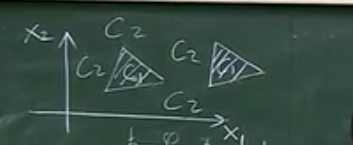2）神经元
神经元在数学模型中的位置如下图所示，神经元的个数其实就是围成决策面的直线条数，围成决策面的条数有多少，那么神经元就有多少个，若是曲线，则有无数个神经元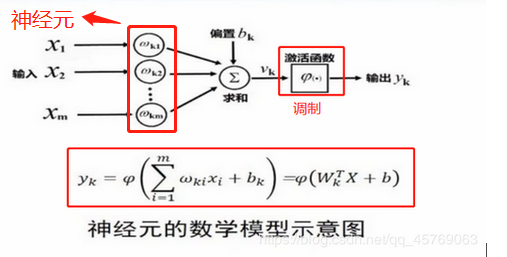3）神经网络层数n
神经网络层数一般由决策面的个数决定，若决策面只有一个，神经网络数学模型采用两层神经网络就可以实现，但是如果决策面有多个（如上面的C1类的区域分开成了两个决策面）则神经网络数学模型采用三层神经网络就可以实现
决策面个数m，则神经网络层数n：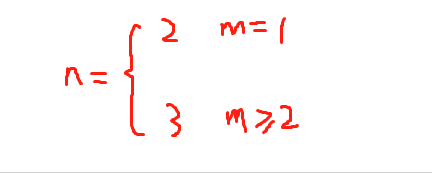所以三层神经网络可以模拟所有的决策面

3、常见的三层神经网络模型（含w,b的参数设置）
1）一个三角形决策面的神经网络模型（两层神经网络）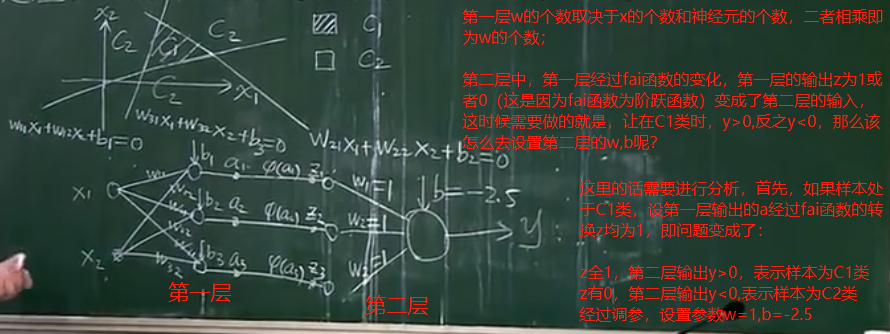2）一个四边形决策面的神经网络模型（两层神经网络）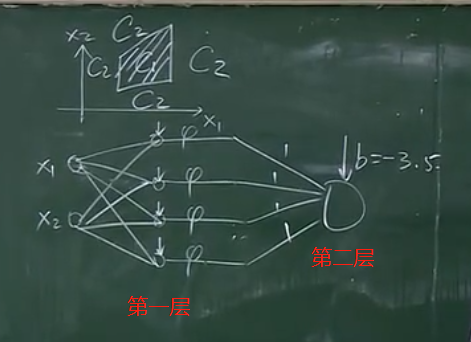3）一个曲线围成决策面的神经网络模型（两层神经网络）
将曲线用无数个非常短的线去替代曲线，只是神经元为无限个

小结1：一个决策面时最后一层常用w,b参数的设置
答：设决策面为1个，围成决策面的直线条数为n，则取：所有w = 1 ; b = - n + 0.5
只有一个决策面的数学模型最后一层神经网络是与逻辑关系，即所有输入为1，归类为C1，反之归类为C2

4)两个决策面的神经网络模型（三层神经网络）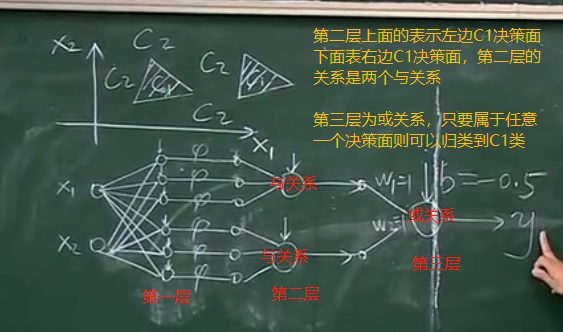一个决策面的神经元在第二层的输出为一个，有多少个决策面第二层就会有多少个输出（与关系）
5)两个以上决策面的神经网络模型（三层神经网络）
两个以上的决策面的神经网络模型和两个决策面是类似的，只是第一层的神经元数目多了，第二层的与关系多了而已

小结2：多个决策面时最后一层w,b常用参数和第二层w,b常用参数的设置
答：多个决策面时，最后一层的w,b参数固定为：w = 1 , b = -0.5
第二层每个与关系处即每一个决策面的第二层的w,b参数设置参考上面的小结1
疑问：
那么该怎么取利用这个神经网络模型去解决实际的问题呢？至今为止神经网络的理论还不够完善，大部分还是通过实验的方式来获得神经网络模型中的参数（主要是第一层中的w,b参数），也因为这样，神经网络成为了一个实验性的学科，但是也有常用的神经网络模型训练方法（训练就是通过训练样本求解参数的一个过程，，进而获得完整的模型，利用模型可以对测试样本进行分类），可以参见文章：《机器学习——人工神经网络之后向传播算法（BP算法）》
展开全文• 论文研究-多层细胞神经网络模型的一般状态解及动力值域估计.pdf, 针对多层细胞神经网络模型，分析了其一般状态解并对其动力值域作了估计。
• 在感知器神经网络模型与线性神经网络模型学习算法中，理想输出与实际输出之差...1985年Rumelhart、McClelland提出了BP网络的误差反向后传（BP）学习算法，实现了Minsky设想的多层神经网络模型。BP算法在于利用输出后的
在感知器神经网络模型与线性神经网络模型学习算法中，理想输出与实际输出之差被用来估计神经元连接权值误差。当解决线性不可分问题而引入多级网络后，如何估计网络隐含层神经元的误差就成了一大难题。因为在实际中，无法知道隐含层的任何神经元的理想输出值。1985年Rumelhart、McClelland提出了BP网络的误差反向后传（BP）学习算法，实现了Minsky设想的多层神经网络模型。
BP算法在于利用输出后的误差来估计输出层的直接前导层的误差，再用这个误差估计更前一层的误差，如此一层一层的反传下去，就获得了所有其他各层的误差估计。使用BP算法进行学习的多级肺循环网络称为BP网络，属于前向神经网络类型。虽然这种误差估计本身的精度会随着误差本身“向后传播”而不断降低，但它还是给多层网络的训练提供了比较有效的办法，加之多层前向神经网络能逼近任意非线性函数。
BP神经网络模型
构成BP网络的神经元仍然是神经元。按照BP算法的要求，这些神经元所用的激活函数必须处处可导。一般都使用S型函数。对一个神经元来说，它的网络输入可以表示为：

net=x→⋅ω→

net = \overrightarrow x \cdot \overrightarrow \omega

其中

x→
$\overrightarrow x$ 表示该神经元所接受的输入，

ω→
$\overrightarrow \omega$ 表示神经元对应的连接权值。
该神经元的输出为：

y=f(net)=11+e−net

y = f(net) = \frac{1}{1 + e^{-net}}

进一步地，我们可以求y关于net的导数：

f′(net)=e−net(1+e−net)2=1+e−net−1(1+e−net)2=11+e−net−1(1+e−net)2=y(1−y)

\begin{align}f'(net) & = \frac{e^{-net}}{(1 + e^{-net})^2} \\& = \frac{1 + e^{-net} - 1}{(1 + e^{-net})^2} \\& = \frac{1}{1 + e^{-net}} - \frac{1}{(1 + e^{-net})^2} \\& = y(1 - y) \end{align}

显然地 我们可以注意到

limnet→+∞11+e−net=1limnet→−∞1(1+e−net)2=0

\lim_{net \to + \infty}\frac{1}{1 + e^{-net}} = 1 \\ \lim_{net \to - \infty}\frac{1}{(1 + e^{-net})^2} = 0

根据S型激活函数可知，y的值域为(0, 1)， 从而，f’(net)的值域为(0, 0.25)，而且是在y=0.5时，f’(x)有最大值。
BP网络的标准学习算法
标准BP算法是基于梯度下降法的学习算法，学习过程是通过调整权值和阈值，使删除期望值和神经网络实际输出值的均方误差区域最小而实现的，但是它只用到均方误差函数对权值和阈值的一阶导数（梯度）的信息，使得算法存在收敛速度缓慢、容易陷入局部极小等缺陷。
定义：  * 输入向量

x→
$\overrightarrow x$  * 隐含层输入向量

hi→
$\overrightarrow{hi}$  * 隐含层输出向量

ho−→
$\overrightarrow{ho}$  * 输出层输入向量

yi→
$\overrightarrow{yi}$  * 输出层输出向量

yo→
$\overrightarrow{yo}$  * 期望输出向量

d→
$\overrightarrow d$  * 输入层与隐含层的连接权值

ωih
$\omega_{ih}$  * 隐含层与输出层的连接权值

ωho
$\omega_{ho}$  * 隐含层各神经元的阈值

bh
$b_h$  * 输出层各神经元的阈值

bo
$b_o$  * 样本数据个数

k
$k$
* 激活函数 f(⋅)$f(\cdot)$
BP标准算法具体实现步骤如下：  1. 网络初始化，给

wih
$w_{ih}$

who
$w_{ho}$

bh
$b_h$

bo
$b_o$ 分别赋一个区间(-1, 1)内的随机数，并设定误差函数为

e=12∑o=1q(do(k)−yo(k))2

e = \frac{1}{2} \sum^q_{o =1}(d_o(k) - y_o(k))^2给定计算精度值

ε
$\varepsilon$ 和最大学习次数M
2. 随机选取第k个输入样本

x(k)−→−
$\overrightarrow{ x(k)}$ 以及对应的期望输出

d(k)−→−
$\overrightarrow{d(k)}$
3. 计算隐含层各神经元的输入

hih(k)
$hi_h(k)$ ,然后用输入以及激活函数计算隐含层各神经元的输出

hoh(k)
$ho_h(k)$

hih(k)=∑inwihxi(k)−bhhoh(k)=f(hih(k))yio(k)=∑hpwhohoh(k)−boyoo(k)=f(yio(k))

\begin{align}& hi_h(k) = \sum^n_i w_{ih}x_i(k) - b_h \\& ho_h(k) = f(hi_h(k)) \\& yi_o(k) = \sum^p_hw_{ho}ho_h(k) - b_o\\& yo_o(k) = f(yi_o(k)) \end{align}
4. 利用网络期望输出向量

d(k)−→−
$\overrightarrow{d(k)}$ 网络的实际输出

yoo(k)
$yo_o(k)$ ，计算误差函数对输出层各神经元的偏导数

δo(k)
$\delta_o(k)$

δo(k)=(do(k)−yoo(k))yoo(k)(1−yoo(k))

\delta_o(k) = \left( d_o(k) - yo_o(k) \right)yo_o(k)(1-yo_o(k))
5. 利用隐含层到输出曾的连接权值

who(k)
$w_{ho}(k)$ 、输出层的

δo(k)
$\delta_o(k)$ 和隐含层的输出

hoh(k)
$ho_h(k)$ 计算误差函数对隐含层各神经元的偏导数

δh(k)
$\delta_h(k)$

δh(k)=[∑o=1qδo(k)who]hoh(k)(1−hoh(k))

\delta_h(k) = \left[ \sum^q_{o=1} \delta_o(k) w_{ho}\right]ho_h(k)(1-ho_h(k))
6. 利用输出层各神经元的

δo(k)
$\delta_o(k)$ 和隐含层各神经元的输出

hoh(k)
$ho_h(k)$ 来修正连接权值

who(k)
$w_{ho}(k)$ 和阈值

bo(k)
$b_o(k)$ :

wN+1ho(k)=wNho(k)+ηδo(k)hoh(k)bN+1o(k)=bNo(k)+ηδo(k)

w^{N+1}_{ho}(k) = w^N_{ho}(k) + \eta \delta_o(k)ho_h(k) \\ b^{N+1}_o(k) = b_o^N(k) + \eta \delta_o(k)
N为调整前，N+1为调整后，

η
$\eta$ 为学习率， 在(0, 1) 之间取值。
7. 使用隐含层各神经元的

δh(k)
$\delta_h(k)$ 和输入层各神经元的输入

xi(k)
$x_i(k)$ 修正连接权和阈值

wN+1ih=wNih+ηδh(k)xi(k)bN+1h(k)=bN+1h(k)+ηδh(k)

w^{N+1}_{ih} = w^N_{ih} + \eta \delta_h(k)x_i(k) \\ b_h^{N+1}(k) = b^{N+1}_{h}(k) + \eta \delta_h(k)
8. 计算全局误差E

E=12m∑k=1m∑o=1q(do(k)−yo(k))2

E = \frac{1}{2m} \sum^m_{k=1} \sum^q_{o=1}(d_o(k) - y_o(k))^2
9. 判断网络误差是否满足要求，当

E<ε
$E < \varepsilon$ 或学习次数大于设定的最大次数M，则算法结束。否则，随机选取下一个学习样本及对应年的期望输出，返回到第三步，进入下一轮的学习过程。

展开全文• 1. 多层人工神经网络模型 由输入层、隐藏层（可有多层）和输出层组成。神经网络中最基本的成分是神经元（neuron）。其中，输入层神经元仅接收外界输入，不进行函数处理；隐藏层和输出层则包含功能神经元，能将接收...
1. 多层人工神经网络模型
多层感知机MLP（Multi-Layer Perceptron）也叫多层人工神经网络。一般的，常见的神经网络是如下图所示的层级结构。由输入层、隐藏层（可有多层）和输出层组成。神经网络中最基本的成分是神经元（neuron）。每层神经元与下一层神经元全互连，神经元之间不存在同层连接，也不存在跨层连接。这样的神经网络结构通常称为“多层前馈神经网络”（multi-layer feedforward neural networks)，（前馈并不意味着网络中信号不能向后传，而是指网络拓扑结构上不存在环或回路），其中，输入层神经元仅接收外界输入，不进行函数处理；隐藏层和输出层则包含功能神经元，能将接收到的总输入值与一定的阈值进行比较，然后通过“激活函数”（activation function）处理以产生神经元的输出。若将阈值也作为输入信号在神经网络中标出，则除输出层之外，各层会多出一个固定输入为-1的“哑结点”（dummy node），该节点与下一层的连接权即为阈值。这样权重和阈值的学习就可以统一为权重的学习。为方便后续解释，下图中并未标出哑结点。  下图给出“单隐层网络”模型并解释各层功能及其作用方式：输入层拥有d个神经元，代表输入样本在d个属性下的值； 输入层信号通过带权重的连接传递至隐藏层，隐藏层神经元分别接收到各自的总输入值ah(h=1,2…,q)，将其与神经元的阈值进行比较，然后通过“激活函数”处理产生相应的输出bh(h=1,2,…,q)； 隐藏层输出信号同样通过带权重的连接传递至输出层，输出层神经元分别接收各自的总输入值co(o=1,2,…,l)，并通过激活函数处理产生最后输出y’o(o=1,2,…l)，即对该样本x的预测y’（这里的y’为 l 维列向量）。输出层神经元代表分类结果，其个数取决于最终分类标签的个数。 神经网络的学习过程，就是根据训练数据来调整神经元之间的“连接权”（connection weight）以及每个功能神经元的阈值；换言之，神经网络“学”到的东西，蕴涵在连接权与阈值中。  为了方便理解，这里举出该神经网络模型的应用实例：识别手写数字。 假设样本为n个手写数字图像，每个数字图像由28×28像素组成，每个像素由灰度值表示。我们把28×28的像素展开变成一个有784个维度的一维行向量，即一个样本向量，那么此时输入层的神经元个数就会有784个，即图中的d=784。因为是识别单个手写数字，其结果会有0-9这十种情况，因此输出层就会有10个神经元，即图中的 l=10。至于隐藏层的层数以及单元数则作为要优化的额外超参数，可以通过交叉验证技术解决，在此不对其进行详细展开。  关于激活函数： 理想的激活函数是如下图（a)所示的阶跃函数，它将输入值映射为输出值“0”或“1”，显然“1”对应于神经元兴奋，“0”对应于神经元抑制。然而，阶跃函数具有不连续、不光滑等不太好的性质，因此实际常用Sigmoid函数作为激活函数，如下图(b)所示。有时，输出层神经元也采用线性激活函数，即直接以输入值c与阈值θ的比较结果作为最后的预测值y’输出。2. 算法公式推导
（1）正向传播（Forward Propagation）： 利用正向传播激活神经网络
接下来，我们利用上述的神经网络模型，进一步用数学表达式来详细解释神经网络如何通过正向传播从输入信号输出预测结果的各个步骤： 首先给定数据集 D：即数据集D共有n个样本，且输入样本x(i)由 d 个属性描述，输出 l 维实值向量 y(i),如下所示：将输入样本用矩阵X表示，输出值用矩阵Y表示：上标代表样本标号，下标代表相应的属性维度及分类维度。在输入层输入某一样本在各个属性维度下的值xk(k=1,2,…,d)作为接收信号并通过带权重v的连接传递至隐藏层，成为隐藏层的输入值ah(h=1,2,…q)。下图给出隐藏层的一个神经元如何接收输入层的输出信号：用公式表示为：则对应某一样本的隐藏层输入向量为：记这里的权重矩阵为V：这会是我们之后要学习的连接权之一。 同理可以写出隐藏层的输入值矩阵A：接下来，隐藏层的各个功能神经元将接收到的输入值ah(h=1,2,…,q)，与某一阈值γh(h=1,2,…,q)进行比较，然后通过“激活函数”（这里用sigmoid函数）处理产生神经元的输出bh(h=1,2,…,q)，用公式表示为：则对应某一样本的输出层输出向量为：其中阈值γ也是我们之后要学习的参数。 同样可以写出隐藏层输出值矩阵B：之后，隐藏层神经元的输出bh(h=1,2,…,q)继续通过带权重的连接w传递至输出层，成为输出层的输入值co(o=1,2,…,l)，下图同样给出输出层的一个神经元如何接收隐藏层的输出信号：用公式表示为：则对应某一样本的输入层输入向量为：记这里的权重矩阵为W：这也是我们之后要学习的连接权之一。 同理可以写出输出层的输入值矩阵C：最后，输入层的各个功能神经元将接收到的输入值co(o=1,2,…,q)，与某一阈值θo(o=1,2,…,q)进行比较，然后通过“激活函数”（这里用sigmoid函数）处理产生神经元的输出y’o(o=1,2,…,q)，用公式表示为：则对应某一样本的输出层输出向量为：这里的阈值θ也是我们之后要学习的系数。同样可以写出输出层输出值矩阵Y’：若输出层神经元采用线性激活函数，则输出预测结果y’o(o=1,2,…,q)，用公式表示为：则对应某一样本的输出层输出向量为：以及输出层的输出值矩阵Y’：以上就是“单隐层神经网络”中输入信号如何通过各层的处理最后输出预测结果的正向传播激活网络的全过程。这里将其简单总结如下图所示：（2）逆向传播（BackPropagation，简称BP）： 利用逆向传播学习神经网络的权重系数及阈值BP是一种迭代学习算法，在迭代的每一轮中采用广义的感知机（perceptron）学习规则对参数进行更新估计，即任意参数w的更新方式为：其中Δw是所谓“目标函数”（objective function）关于该参数的梯度（偏导）。若要使目标函数的迭代值与迭代数成反比，即随着迭代的进行，目标函数值越来越小，则目标函数要为凹函数拥有全局最小值用于收敛并采用负梯度下降更新权重；反之，若要使目标函数的迭代值与迭代数成正比，则目标函数为凸函数具有全局最大值同样用于收敛并用正梯度上升更新权重。具体如下图所示：BP算法采用误差函数J(v,w,γ,θ)为目标函数，又称为“代价函数”（cost function），其数学表达式为：既为误差函数，则必然要求其值随着迭代的进行而下降，从而找到能使代价函数为全局最小值的权重，因此代价函数为凹函数，采用梯度下降（gradient descent）策略，则Δw可以表示为：其中，η为学习率，控制梯度下降的步长。当学习率很小时会使收敛缓慢，学习率过大则会增加忽略全局最小值的可能性。因此学习率η也是算法在实际运用中需要调优的超参数。 此外需要注意的一点是，多层神经网络的代价函数与自适应线性神经元（Adaline）以及逻辑回归（logistic regression）这样的单层网络的代价函数相比更为复杂。单层网络的代价函数与参数相关的误差表面就如同上图所示一样通常是光滑的、无突起的。而多层神经网络的代价函数是复杂嵌套函数，维度更高，其误差表面有许多突起，即拥有许多局部极小值，如下图所示：因此，要想找到全局最小值就必须克服这些局部极小值。神经网络参数化通常所面临的挑战就是处理高维特征空间的大量权重系数。 微积分中的链式规则就是一种计算复杂嵌套函数导数的方法，如 F(x)=f(g(h(u(v(x))))) 应用链式法则的求导为：计算机代数已经开发出了一套非常有效地解决这类问题的技术，也就是所谓的自动微分。自动微分有正向和反向两种模式，反向传播仅仅是反向模式自动微分的特例。关键是正向模式应用链式法则可能相当昂贵，因为要与每层的大矩阵（雅可比矩阵）相乘，最终乘以一个向量以获得输出。反向模式的技巧是从右往左：用一个矩阵乘以一个向量，从而产生另一个向量，然后再乘以下一个矩阵，以此类推。矩阵向量乘法比矩阵矩阵乘法在计算成本上要便宜得多，这就是为什么反向传播算法是神经网络训练中最常用的算法之一。 根据上述分析，本质上可以认为BP算法是一种计算多层神经网络复杂成本函数的偏导数的非常有效的方法，其目标是利用这些导数来学习权重系数，以实现多层人工神经网络的参数化。 接下来我们开始利用微积分的链式规则来逐步推导BP算法中的权重更新公式，以上述所介绍的“单隐层神经网络”模型为例。 首先，这里我们需要更新的权重系数有四个，它们分别是：两个权重矩阵V、W，以及两组阈值向量γ和θ，我们对它们采用如下所示的广义感知机学习规则以及负梯度下降进行迭代更新：从神经网络的右往左对代价函数J(v,w,γ,θ）进行求导，首先会遇到的是隐藏层的权重矩阵W以及阈值向量θ。这里首先写出代价函数J(v,w,γ,θ)的完整嵌套函数形式：根据上式可写出代价函数关于权重w以及阈值θ的负梯度：其中：值得一提的是，有些多层神经网络的输出层使用的激活函数为线性激活函数，而非Sigmoid函数，此时，权重w以及阈值θ的偏导则为：其中：若设输出层的误差δ1o(o=1,2,…,l)为：或者当输出层神经元的激活函数为线性激活函数时δo(o=1,2,…,l)可表示为：则Δw和Δθ可以表示为：接下来，继续从右往左求导，得到权重v和阈值γ的负梯度：其中：若输出层神经元的激活函数为线性激活函数，则权重v和阈值γ的负梯度可表示为：其中：若设隐藏层的误差δ2h(h=1,2,…,q)为：其中δ1o(o=1,2,…,l)为：或者当输出层神经元的激活函数为线性激活函数时δ2h(h=1,2,…,q)可表示为：其中δ1o(o=1,2,…,l)为：则Δv和Δγ可以表示为：至此推导出所有参数的负梯度，将各个参数的权重更新总结如下：其中：用矩阵表示为：其中：3. 关于算法的实际应用
关于每次参数更新所针对的数据集大小对模型学习的影响： 在实际应用中，一般来说标准BP算法每次更新只针对单个样例，即上述推导过程中的样本个数n=1的情况。在这种情况下，参数更新得非常频繁，而且对不同样例进行更新的效果可能出现“抵消”现象。 与之相对应的累积BP算法直接针对整体训练集的总误差，即n>>1的情况。在这种情况下，每更新一次权重系数，算法都要读取整个训练集的数据一遍，因此其参数更新频率会非常缓慢。 在实际应用中，我们可以采取折中的方式，即采用小批量（mini-batch）的数据读取方式，每次只读取总训练数据的一小部分数据来更新参数。这样既可以提高计算效率，也能够更快地进行参数更新。  关于BP神经网络的“过拟合”（overfitting）问题： 除此之外，多层神经网络由于其强大的表示能力而经常遭遇“过拟合”（overfitting），即模型在训练集上表现良好但无法概括未见过的新数据或测试数据的情况。在这种情况下，我们也称模型具有”高方差“（high variance）的属性，即模型对训练数据的随机性很敏感。 有两种策略常用来缓解BP网络的过拟合问题： 第一种策略是"早停"（early stopping）：将数据分成训练集和验证集，训练集用来计算梯度、更新权重和阈值，验证集用来估计误差。若训练集误差降低但验证集误差升高，则停止训练，同时返回具有最小验证集误差的连接权和阈值。 第二种策略是"正则化"（regularization）：其基本思想是在误差目标函数中增加一个用于描述网络复杂度的部分，例如连接权和阈值的平方和（L2正则化）。由于过拟合问题通常是由于训练后的模型表达式过于复杂所导致的，因此通过调整正则项系数λ使该正则项经过微分后能够不同程度地降低各个权重系数值以减少各个特征对结果的影响从而使模型得到不同程度的简化，就能最终达到抵抗模型过拟合的效果。
4. 参考文献
 周志华. 机器学习(第1版)[M]. 出版地:北京市清华大学学研大厦A座, 出版单位:清华大学出版社, 出版年份:2016, 起止页码:97-106. Sebastian Raschka，Vahid Mirijalili. Python机器学习(第2版)[M]. 出版地:北京市西城区百万庄大街22号, 出版单位:机械工业出版社, 出版年份:2019, 起止页码:238-261.
展开全文机器学习
• Pytorch Note12 多层神经网络 文章目录Pytorch Note12 多层神经网络多层神经网络理解神经网络sigmoid 激活函数tanh 激活函数ReLU 激活函数神经网络的结构为什么要使用激活函数二分类问题决策边界随机生成数据...python 深度学习
• ## 一文看懂25个神经网络模型

万次阅读 多人点赞 2017-06-17 10:26:08
光是知道各式各样的神经网络模型缩写（如：DCIGN、BiLSTM、DCGAN……还有哪些？)，就已经让人招架不住了。因此，这里整理出一份清单来梳理所有这些架构。其中大部分是人工神经网络，也有一些完全不同的怪物。尽管...深度学习 LSTM 深度信念网络
• ## 多层神经网络

千次阅读 2018-04-25 10:03:10
《python machine learning 》chapter 12 Implementing a Multilayer Artificial Neural Network from Scratch【主要内容】（1）总体理解多层神经网络（2）从头开始实施神经网络训练的基本反向传播算法（3）训练...
• BP（Back Propagation）网络是1986年由Rumelhart和McCelland为首的科学家小组提出，是一种按误差逆传播算法训练的多层前馈网络，是目前应用最广泛的神经网络模型之一。BP网络能学习和存贮大量的输入-输出模式映射...深度学习
• ## 神经网络模型

千次阅读 2015-07-30 17:25:48
什么是神经网络 神经网络（Neural Networks,NN）是由大量的、简单的处理单元（称为神经元）广泛地互相连接而形成的复杂网络系统，它反映了人脑功能的许多基本特征，是一个高度复杂的非线性动力学习系统。神经网络...人工智能 算法
• 简单的神经网络模型是：前向回馈神经网络（feed-forward）, 其包括以下几层： 输入、多个层次的对输入的回馈以及最终的输出。 简单的神经网络的训练过程包括以下几个步骤： 定义一个包含多个可学习参数（权重）的...Pytorch 反向传播
• P神经元1.2 激励函数1.2.1 单位阶跃函数1.2.2 logistic函数（sigmoid）1.2.3 tanh函数（双曲正切函数）1.2.4 ReLU（修正线性单元）1.2.5 激励函数对比1.3 罗森布拉特感知器1.4 Adaline（自适应线性神经元）二、神经...
• 提出了有理式多层前馈神经网络的数学模型, 给出了有理式多层神经网络的学习算法. 就计算复杂度而 言, 有理式神经网络的学习算法与传统的多层神经网络反传播算法是同阶的. 函数逼近应用实例结果表明, 将有理式...
• "BP（Back Propagation）网络是1986年由Rumelhart和McCelland为首的科学家小组提出，是一种按误差逆传播算法训练的多层前馈网络，是目前应用最广泛的神经网络模型之一。BP网络能学习和存贮大量的输入-输出模式映射...机器学习 决策树 激活函数
• ## 多层神经网络ANN

千次阅读 2016-08-14 22:32:23
本文基于 cnblog学习总结，基于的博客地址如下： http://www.cnblogs.com/ronny/p/ann_02.html在实际应用过程中...多层神经网络是由多个层结构组成的网络系统，他的每一层都是若干个神经元结点构成，该层的任意一个结点
• 3.3 感知器的局限性 3.4 单层感知器神经网络的MATLAB仿真程序设计 3.5 多层感知器神经网络及其MATLAB仿真 3.6 感知器应用于线性分类问题的进一步讨论 第4章 线性神经网络 4.1 线性神经网络模型 4.2 线性神经网络的...
• 仅使用Numpy实现的多层全连接神经网络，学习过程中产生的系列代码AI Numpy
• 卷积神经网络（CNN）是一种特殊的深层的神经网络，广泛应用于视觉图像识别领域。卷积神经网络（CNN）将人工神经网络和深度学习技术相结合而产生的一种新型人工神经网络方法，具有局部感受区域、层次结构化、特征提取...卷积神经网络 深度学习
• 推导前向多层神经网络的学习算法，以及BP学习算法中几个值得注意的问题，设计网络学习过程的步骤
• ## BP神经网络模型及梯度下降法

万次阅读 多人点赞 2017-06-26 23:44:38
BP（Back Propagation）网络是1985年由Rumelhart和McCelland为首的科学家小组提出，是一种按误差逆传播算法训练的多层前馈网络，是目前应用最广泛的神经网络模型之一。 BP网络能学习和存贮大量的输入-输出模式映射...梯度下降法
• 第七讲 人工神经网络模型 随着人工神经网络应用研究酌不断深入新的神经网络 模型不断推出现有的神经网络棋型已达近百种在智 能控制领域中应用最多的是以下几种基本模型和它们 的改进型即前向多层感知器(BP网络)...
• 作者：Bipolar Bear ... 来源：知乎 著作权归作者所有。商业转载请联系作者获得授权，非商业转载请注明出处。 ...以我的理解，两种网络被设计出来，所要解决的...多层神经网络与universal approximation theorem...